Properties of Gases & Gas Mixtures

# Properties of Gases & Gas Mixtures - Notes | Study Mechanical Engineering SSC JE (Technical) - Mechanical Engineering

 1 Crore+ students have signed up on EduRev. Have you?

PROPERTIES OF GASES AND GAS MIXTURE

• A hypotetical gas which obeys ideal gas equation at all pressures and temperature is called an ideal gas.
•  At very low pressure and high temperature, real gas approaches the ideal gas behaviour.
•  Forms of ideal gas equation
•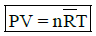Universal gas constant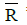= 8.3143 kJ/kg mol K

n = number of moles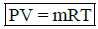m is mass of the gas                    m = nM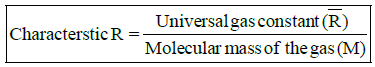Rair = 0.287 kJ/kg K
Mair = 28.96

• PV = NkT
N = Number of molecules of gas
k = Boltzmann constant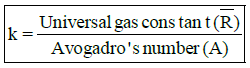•, k are constants while R is variable which depends upon the molecular mass of the gas.
•  Cp – Cv = R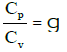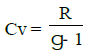and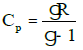•  Values of g for different gases.
 Gases                  Monatomic gases  Diatomic gasesPolyatomic gases  Air value of 5/3 7/5 4/3 1.41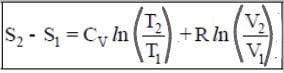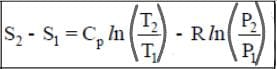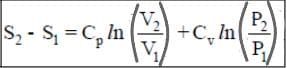• Polytropic process (PVn = Constant)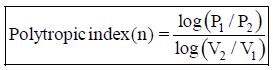• Van der Waals equation for real gas
•  Assumptions for ideal gas:
•  There is no force among the molecule of an ideal gas.
•  Volume of gas molecule is negligible as compared to the volume of gas container.
•  At very high pressure and low temperature both the assumptions go wrong. So for real gases, vander waals equation is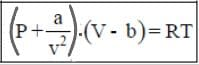The Ideal Gas Law is based on the assumptions that gases are composed of point masses that undergo perfectly elastic collisions. However,real gases deviate from those assumptions at low temperatures or high pressures.  Imagine a container where the

pressure is increased.  As the pressure increases, the volume of the container decreases. The volume occupied by the gas particles is no longer negligible compared to the volume of the container and the volume of the gas particles needs to be taken into account.  At low temperatures, the gas particles have lower kinetic energy and do not move as fast.  The gas particles are affected by the intermolecular forces acting on them, which leads to inelastic collisions between them.  This leads to fewer collisions with the container and a lower pressure than what is expected from an ideal gas.

Cohesive forces are the intermolecular forces (such as those from hydrogen bonding and Van der Waals forces) which cause a tendency in liquids to resist separation. These attractive forces exist between molecules of the same substance. For instance, rain falls in droplets, rather than a fine mist, because water has strong cohesion which pulls its molecules tightly together, forming droplets. This force tends to unite molecules of a liquid, gathering them into relatively large clusters due to the molecules' dislike for its surrounding.

Adhesive forces are the attractive forces between unlike molecules. They are caused by forces acting between two substances, such as mechanical forces (sticking together) and electrostatic forces (attraction due to opposing charges). In the case of a liquid wetting agent, adhesion causes the liquid to cling to the surface on which it rests. When water is poured on clean glass, it tends to spread, forming a thin, uniform film over the glasses surface. This is because the adhesive forces between water and glass are strong enough to pull the water molecules out of their spherical formation and hold them against the surface of the glass, thus avoiding the repulsion between like molecules.

•  Relation among a, b, R, Vc, Tc, Pc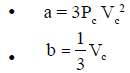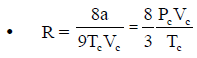a, b = vander waals constant

Pc, Vc, Tc = critical pressure volume and temperature of the gas

•  Boyle's temperature (TB)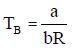• Dalton's law of partial pressures

For homogenous mixture of ideal gases in a container, if P1, P2, P3... are pressure for the gases when they remain alone in the container. The total pressure in the container at the same temperature is

•  P = P1 + P2 + P3 + .....
•  n = n1 + n2 + n3 + .....
•   x1 = x2 + x3 + .... = 1

n1, n2, n3 ....... = number of moles of gases
x1, x2, x3 ....... = mole fractions of gases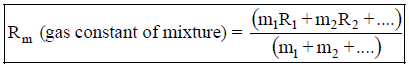The document Properties of Gases & Gas Mixtures - Notes | Study Mechanical Engineering SSC JE (Technical) - Mechanical Engineering is a part of the Mechanical Engineering Course Mechanical Engineering SSC JE (Technical).
All you need of Mechanical Engineering at this link: Mechanical Engineering

## Mechanical Engineering SSC JE (Technical)

6 videos|97 docs|55 tests

## Mechanical Engineering SSC JE (Technical)

6 videos|97 docs|55 tests

Track your progress, build streaks, highlight & save important lessons and more!

,

,

,

,

,

,

,

,

,

,

,

,

,

,

,

,

,

,

,

,

,

;